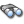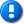alvinalexander.com | career | drupal | java | mac | mysql | perl | scala | uml | unix

# Commons Math example source code file (optimization.xml)

This example Commons Math source code file (optimization.xml) is included in the DevDaily.com "Java Source Code Warehouse" project. The intent of this project is to help you "Learn Java by Example" TM.

## Java - Commons Math tags/keywords

a, direct, in, in, it, license, license, multivariaterealoptimizer, the, the, this, this, users, when

## The Commons Math optimization.xml source code

```<?xml version="1.0"?>

<!--
Licensed to the Apache Software Foundation (ASF) under one or more
contributor license agreements.  See the NOTICE file distributed with
The ASF licenses this file to You under the Apache License, Version 2.0
(the "License"); you may not use this file except in compliance with

Unless required by applicable law or agreed to in writing, software
WITHOUT WARRANTIES OR CONDITIONS OF ANY KIND, either express or implied.
See the License for the specific language governing permissions and
-->

<?xml-stylesheet type="text/xsl" href="./xdoc.xsl"?>
<!-- \$Revision: 799857 \$ \$Date: 2009-08-01 09:07:12 -0400 (Sat, 01 Aug 2009) \$ -->
<document url="optimization.html">

<properties>
<title>The Commons Math User Guide - Optimization
</properties>

<body>
<section name="12 Optimization">
<subsection name="12.1 Overview" href="overview">
<p>
The optimization package provides algorithms to optimize (i.e. either minimize
or maximize) some objective or cost function. The package is split in several
sub-packages dedicated to different kind of functions or algorithms.
<ul>
<li>the univariate package handles univariate scalar functions,
<li>the linear package handles multivariate vector linear functions
with linear constraints,</li>
<li>the direct package handles multivariate scalar functions
using direct search methods (i.e. not using derivatives),</li>
<li>the general package handles multivariate scalar or vector functions
using derivatives.</li>
<li>the fitting package handles curve fitting by univariate real functions
</ul>
</p>
<p>
The top level optimization package provides common interfaces for the optimization
algorithms provided in sub-packages. The main interfaces defines defines optimizers
and convergence checkers. The functions that are optimized by the algorithms provided
by this package and its sub-packages are a subset of the one defined in the
<code>analysis package, namely the real and vector valued functions. These
functions are called objective function here. When the goal is to minimize, the
functions are often called cost function, this name is not used in this package.
</p>
<p>
The type of goal, i.e. minimization or maximization, is defined by the enumerated
<a href="../apidocs/org/apache/commons/math/optimization/GoalType.html">
GoalType</a> which has only two values: `MAXIMIZE` and `MINIMIZE`.
</p>
<p>
Optimizers are the algorithms that will either minimize or maximize, the objective
function by changing its input variables set until an optimal set is found. There
are only four interfaces defining the common behavior of optimizers, one for each
supported type of objective function:
<ul>
<li>
UnivariateRealOptimizer</a> for
<li>
MultivariateRealOptimizer</a> for
<li>
DifferentiableMultivariateRealOptimizer</a> for
<li>
DifferentiableMultivariateVectorialOptimizer</a> for
</ul>
</p>

<p>
Despite there are only four types of supported optimizers, it is possible to optimize
a transform a <a
href="../apidocs/org/apache/commons/math/analysis/MultivariateVectorialFunction.html">
non-differentiable multivariate vectorial function</a> by converting it to a .
</p>
<p>
These algorithms usage is very similar to root-finding algorithms usage explained
in the analysis package. The main difference is that the <code>solve methods in root
finding algorithms is replaced by <code>optimize methods.
</p>
</subsection>
<subsection name="12.3 Linear Programming" href="linear">
<p>
This package provides an implementation of George Dantzig's simplex algorithm
for solving linear optimization problems with linear equality and inequality
constraints.
</p>
</subsection>
<subsection name="12.4 Direct Methods" href="direct">
<p>
Direct search methods only use cost function values, they don't
need derivatives and don't either try to compute approximation of
the derivatives. According to a 1996 paper by Margaret H. Wright
(<a href="http://cm.bell-labs.com/cm/cs/doc/96/4-02.ps.gz">Direct
Search Methods: Once Scorned, Now Respectable</a>), they are used
when either the computation of the derivative is impossible (noisy
functions, unpredictable discontinuities) or difficult (complexity,
computation cost). In the first cases, rather than an optimum, a
<em>not too bad point is desired. In the latter cases, an
optimum is desired but cannot be reasonably found. In all cases
direct search methods can be useful.
</p>
<p>
Simplex-based direct search methods are based on comparison of
the cost function values at the vertices of a simplex (which is a
set of n+1 points in dimension n) that is updated by the algorithms
steps.
</p>
<p>
The instances can be built either in single-start or in
multi-start mode. Multi-start is a traditional way to try to avoid
being trapped in a local minimum and miss the global minimum of a
function. It can also be used to verify the convergence of an
algorithm. In multi-start mode, the <code>minimizesmethod
returns the best minimum found after all starts, and the <code>etMinima
method can be used to retrieve all minima from all starts (including the one
already provided by the <code>minimizes method).
</p>
<p>
The <code>direct package provides two solvers. The first one is the classical
Nelder-Mead</a> method. The second one is Virginia Torczon's
<a href="../apidocs/org/apache/commons/math/optimization/direct/MultiDirectional.html">
multi-directional</a> method.
</p>
</subsection>
<subsection name="12.5 General Case" href="general">
<p>
The general package deals with non-linear vectorial optimization problems when
the partial derivatives of the objective function are available.
</p>
<p>
One important class of estimation problems is weighted least
squares problems. They basically consist in finding the values
for some parameters p<sub>k such that a cost function
J = sum(w<sub>i(mesi - modi)2) is
minimized. The various (target<sub>i - modeli(pk))
terms are called residuals. They represent the deviation between a set of
target values target<sub>i and theoretical values computed from
models model<sub>i depending on free parameters pk.
The w<sub>i factors are weights. One classical use case is when the
target values are experimental observations or measurements.
</p>
<p>
Solving a least-squares problem is finding the free parameters p<sub>k
of the theoretical models such that they are close to the target values, i.e.
when the residual are small.
</p>
<p>
Two optimizers are available in the general package, both devoted to least-squares
problems. The first one is based on the <a
href="../apidocs/org/apache/commons/math/optimization/general/GaussNewtonOptimizer.html">
Gauss-Newton</a> method. The second one is the  method of the optimizer, along with the target and weight arrays,
thus allowing the optimizer to compute the residuals at will. The last parameter to the
<code>estimate method is the point from which the optimizer will start its
search for the optimal point.
</p>
<p>
In addition to least squares solving, the <a
to optimize <a
href="../apidocs/org/apache/commons/math/optimization/DifferentiableMultivariateRealFunction.html">
DifferentiableMultivariateRealFunction</a>. Both the Fletcher-Reeves and the Polak-Ribière
search direction update methods are supported. It is also possible to set up a preconditioner
or to change the line-search algorithm of the inner loop if desired (the default one is a Brent
solver).
</p>
</subsection>
<subsection name="12.6 Curve Fitting" href="fitting">
<p>
The fitting package deals with curve fitting for univariate real functions.
When a univariate real function y = f(x) does depend on some unknown parameters
p<sub>0, p1 ... pn-1, curve fitting can be used to
find these parameters. It does this by <em>fitting the curve so it remains
very close to a set of observed points (x<sub>0, y0),
(x<sub>1, y1) ... (xk-1, yk-1). This
fitting is done by finding the parameters values that minimizes the objective
function sum(y<sub>i-f(xi))2. This is really a least
squares problem.
</p>
<p>
For all provided curve fitters, the operating principle is the same. Users must first
create an instance of the fitter, then add the observed points and once the complete
sample of observed points has been added they must call the <code>fit method
which will compute the parameters that best fit the sample. A weight is associated
with each observed point, this allows to take into account uncertainty on some points
when they come from loosy measurements for example. If no such information exist and
all points should be treated the same, it is safe to put 1.0 as the weight for all points.
</p>
<p>
The <a
href="../apidocs/org/apache/commons/math/optimization/fitting/CurveFitter.html">
CurveFitter</a> class provides curve fitting for general curves. Users must
provide their own implementation of the curve template as a class implementing
the <a
href="../apidocs/org/apache/commons/math/optimization/fitting/ParametricRealFunction.html">
ParametricRealFunction</a> interface and they must provide the initial guess of the
parameters. The more specialized <a
href="../apidocs/org/apache/commons/math/optimization/fitting/PolynomialFitter.html">
PolynomialFitter</a> and

Other Commons Math examples (source code examples)
Here is a short list of links related to this Commons Math optimization.xml source code file:The search pageOther Commons Math source code examples at this package levelClick here to learn more about this project

```
 ... this post is sponsored by my books ...#1 New Release!FP Best Seller